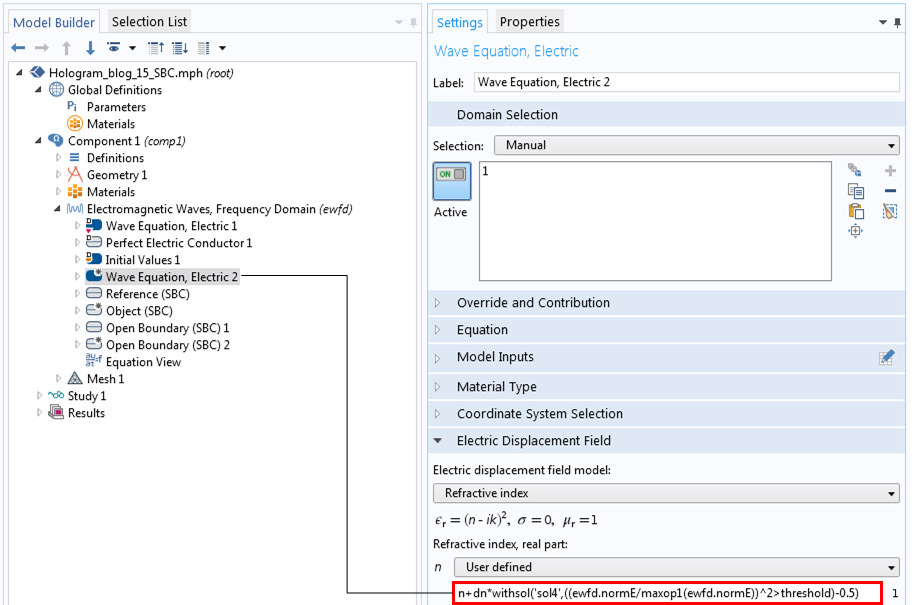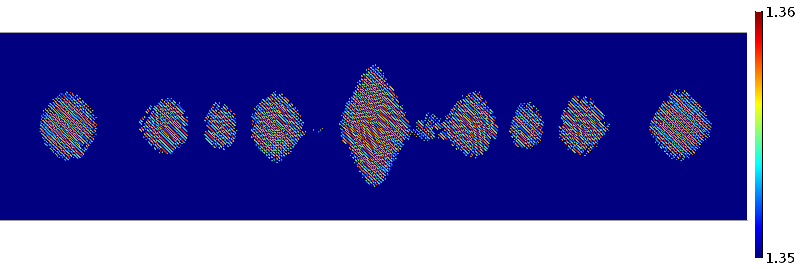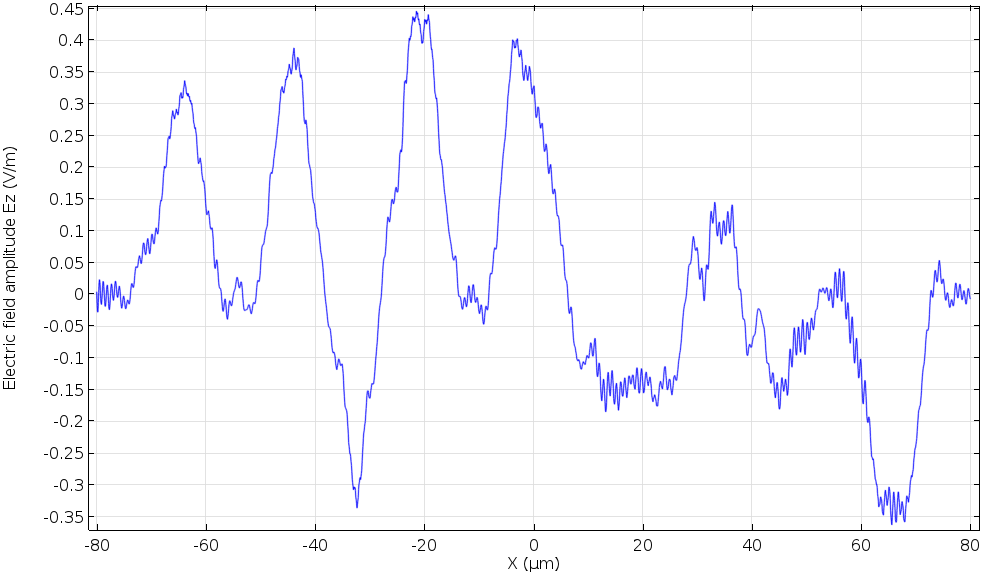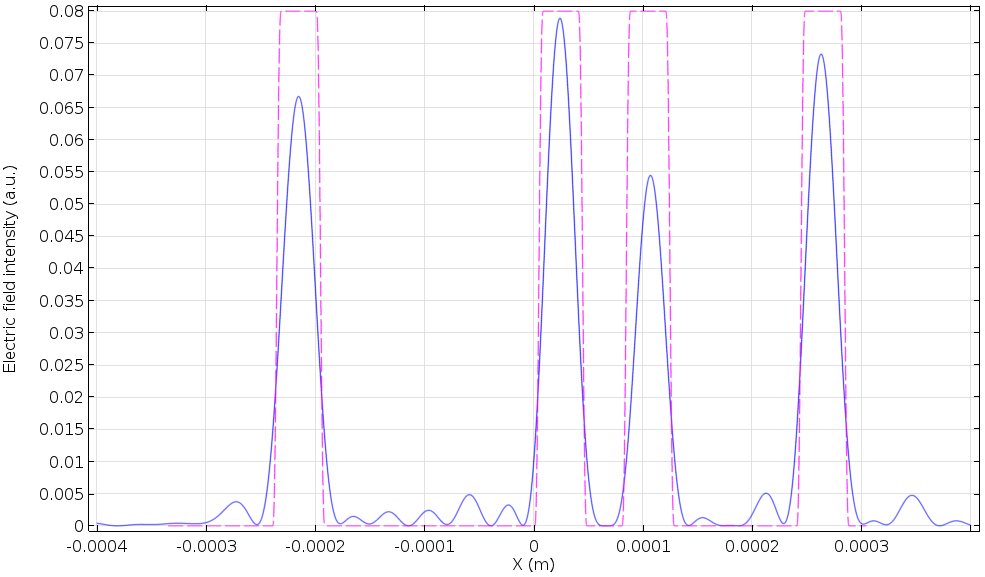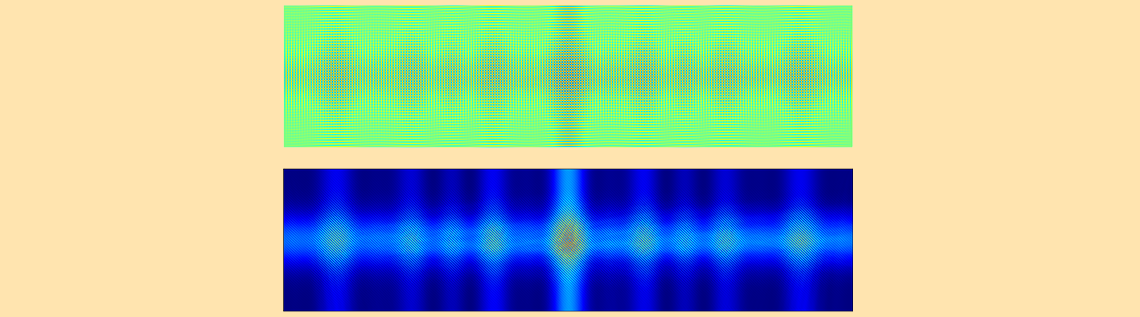# 如何模拟全息页面数据存储系统

2016年 4月 14日

### 全息页面数据存储系统的仿真设计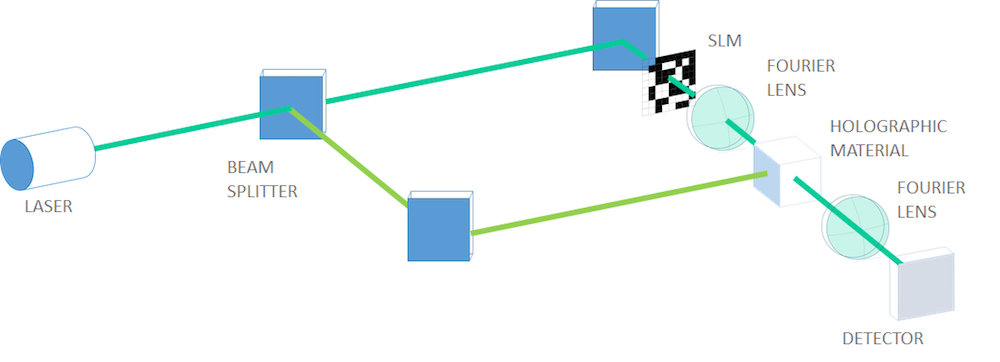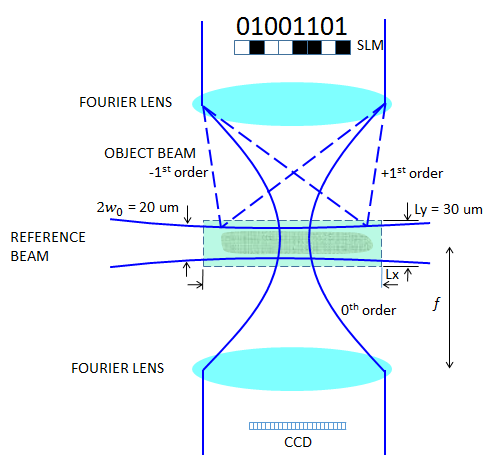#### 定义参考光束

E_z(x,y)=\exp \left (-\frac{y^2} {w_r^2}\right ) \exp \left (-\frac{ink_0 y^2} {2R_r(x)}\right ),

R_r(x)=x \left \{ 1+\left ( \frac{x_R}{x} \right )^2 \right \}.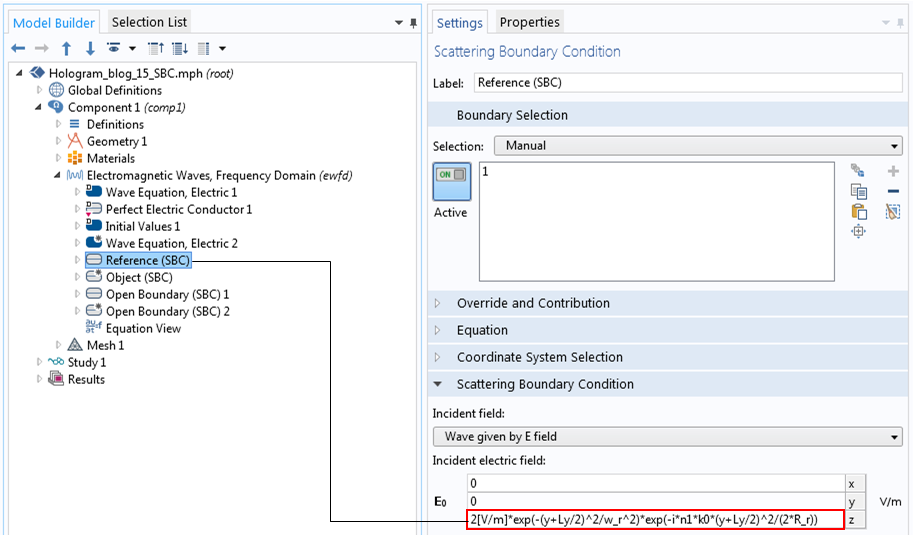#### 定义物体光束

w_0 = \frac{0.61 \lambda_0}{\rm NA},

{\rm NA} = \frac{0.61 \lambda_0} {w_0}

{\rm NA} = \sin \theta \sim \tan \theta = \frac{Nd}{f},

\frac fd = \frac{N}{\rm NA}=\frac{N w_0}{0.61 \lambda_0}.

2d\sin \alpha_1 = \lambda_0,

w_1=f\tan \alpha_1 \sim f\sin \alpha_1=\frac{f \lambda_0}{2d}=\frac{N w_0} {1.22}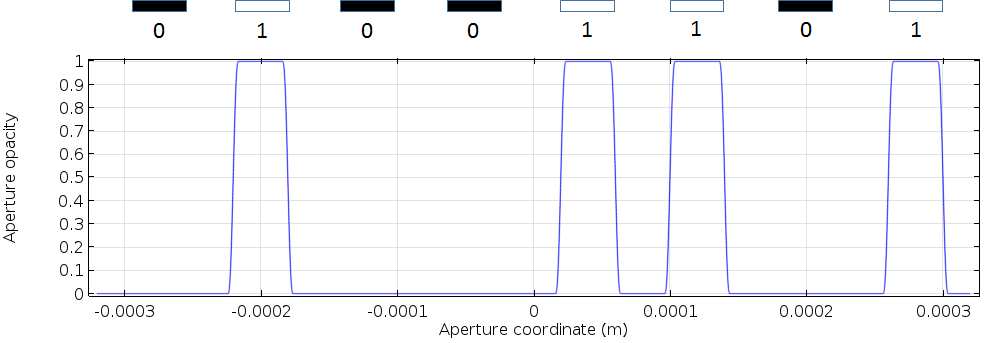SLM 孔径不透明度函数，表示的八位数据 01001101

### 在 COMSOL Multiphysics中实现傅里叶变换

\tilde{E}(u) = \frac{1}{\sqrt{f\lambda_0}}\int_{-\infty}^{\infty}E(x)\exp(- 2 \pi i x u/(f\lambda_0))dx,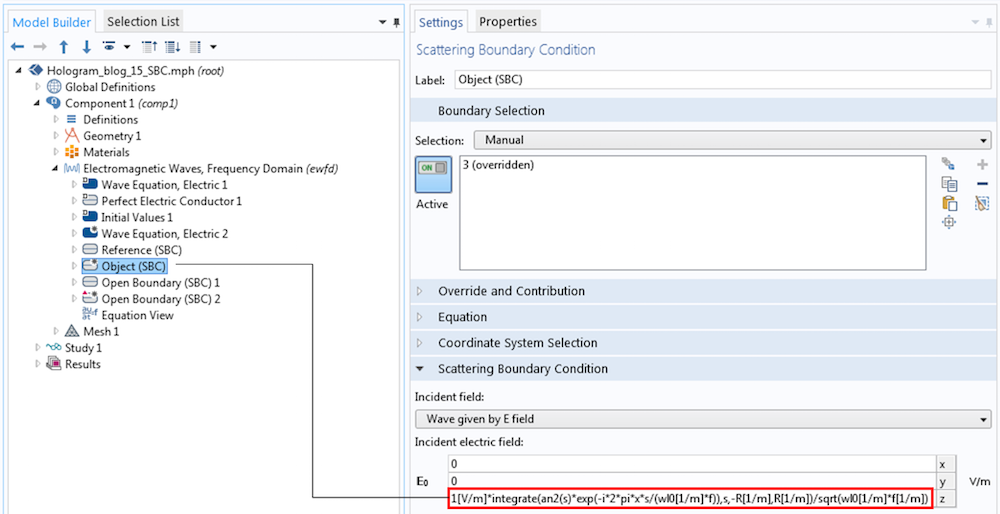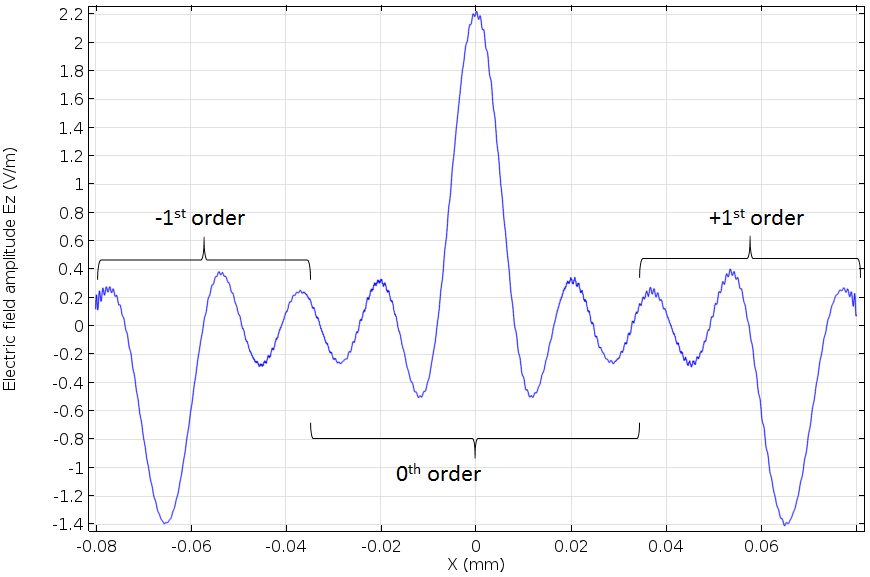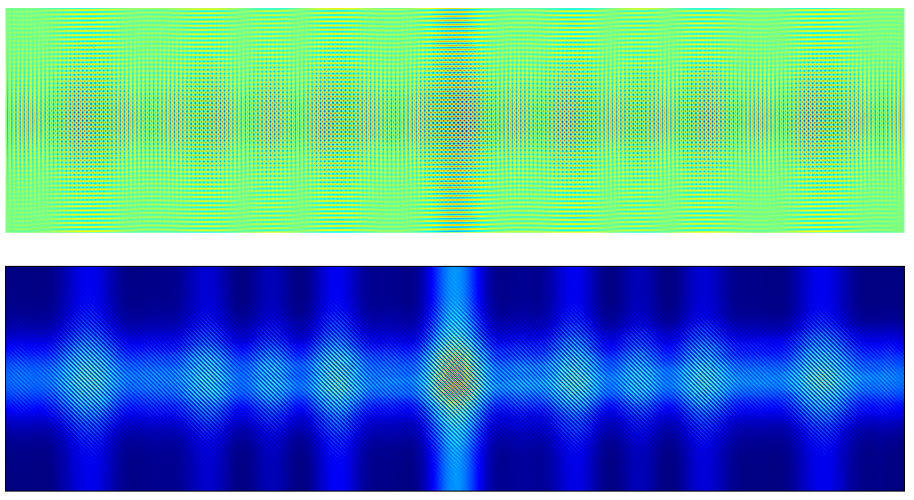### 检索全息数据